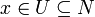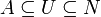# Separation axioms

## Contents

In topology, separation axioms describe classes of topological spaces according to how well the open sets of the topology distinguish between distinct points.

## Terminology

A neighbourhood of a point x in a topological space X is a set N such that x is in the interior of N; that is, there is an open set U such that$x \in U \subseteq N$. A neighbourhood of a set A in X is a set N such that A is contained in the interior of N; that is, there is an open set U such that$A \subseteq U \subseteq N$.

Subsets U and V are separated in X if U is disjoint from the closure of V and V is disjoint from the closure of U.

A Urysohn function for subsets A and B of X is a continuous function f from X to the real unit interval such that f is 0 on A and 1 on B.

## Axioms

A topological space X is

• T0 if for any two distinct points there is an open set which contains just one
• T1 if for any two points x, y there are open sets U and V such that U contains x but not y, and V contains y but not x
• T2 if any two distinct points have disjoint neighbourhoods
• T2½ if distinct points have disjoint closed neighbourhoods
• T3 if a closed set A and a point x not in A have disjoint neighbourhoods
• T3½ if for any closed set A and point x not in A there is a Urysohn function for A and {x}
• T4 if disjoint closed sets have disjoint neighbourhoods
• T5 if separated sets have disjoint neighbourhoods
• Hausdorff is a synonym for T2
• completely Hausdorff is a synonym for T2½
• regular means T0 and T3
• completely regular means T0 and T3½
• Tychonoff means completely regular and T1
• normal means T0 and T4
• completely normal means T1 and T5
• perfectly normal means: normal and such that every closed set is a Gδ

## Properties

• A space is T1 if and only if each point (singleton) forms a closed set.
• Urysohn's Lemma: if A and B are disjoint closed subsets of a T4 space X, there is a Urysohn function for A and B.